# TensorFlow基础¶

• Python基本操作 （赋值、分支及循环语句、使用import导入库）；

• NumPy ，Python下常用的科学计算库。TensorFlow与之结合紧密；

• 向量矩阵 运算（矩阵的加减法、矩阵与向量相乘、矩阵与矩阵相乘、矩阵的转置等。测试题：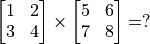）；

• 函数的导数多元函数求导 （测试题：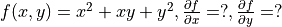）；

• 线性回归

• 梯度下降方法 求函数的局部最小值。

## TensorFlow 1+1¶

import tensorflow as tf


TensorFlow使用 张量 （Tensor）作为数据的基本单位。TensorFlow的张量在概念上等同于多维数组，我们可以使用它来描述数学中的标量（0维数组）、向量（1维数组）、矩阵（2维数组）等各种量，示例如下：

# 定义一个随机数（标量）
random_float = tf.random.uniform(shape=())

# 定义一个有2个元素的零向量
zero_vector = tf.zeros(shape=(2))

# 定义两个2×2的常量矩阵
A = tf.constant([[1., 2.], [3., 4.]])
B = tf.constant([[5., 6.], [7., 8.]])


# 查看矩阵A的形状、类型和值
print(A.shape)      # 输出(2, 2)，即矩阵的长和宽均为2
print(A.dtype)      # 输出<dtype: 'float32'>
print(A.numpy())    # 输出[[1. 2.]
#      [3. 4.]]


TensorFlow的大多数API函数会根据输入的值自动推断张量中元素的类型（一般默认为 tf.float32 ）。不过你也可以通过加入 dtype 参数来自行指定类型，例如 zero_vector = tf.zeros(shape=(2), dtype=tf.int32) 将使得张量中的元素类型均为整数。张量的 numpy() 方法是将张量的值转换为一个NumPy数组。

TensorFlow里有大量的 操作 （Operation），使得我们可以将已有的张量进行运算后得到新的张量。示例如下：

C = tf.add(A, B)    # 计算矩阵A和B的和
D = tf.matmul(A, B) # 计算矩阵A和B的乘积


tf.Tensor(
[[ 6.  8.]
[10. 12.]], shape=(2, 2), dtype=float32)
tf.Tensor(
[[19. 22.]
[43. 50.]], shape=(2, 2), dtype=float32)


## 自动求导机制¶

import tensorflow as tf

x = tf.Variable(initial_value=3.)
y = tf.square(x)


[array([9.], dtype=float32), array([6.], dtype=float32)]


tf.GradientTape() 是一个自动求导的记录器，在其中的变量和计算步骤都会被自动记录。在上面的示例中，变量 x 和计算步骤 y = tf.square(x) 被自动记录，因此可以通过 y_grad = tape.gradient(y, x) 求张量 y 对变量 x 的导数。

X = tf.constant([[1., 2.], [3., 4.]])
y = tf.constant([[1.], [2.]])
w = tf.Variable(initial_value=[[1.], [2.]])
b = tf.Variable(initial_value=1.)
L = 0.5 * tf.reduce_sum(tf.square(tf.matmul(X, w) + b - y))


[62.5, array([[35.],
[50.]], dtype=float32), array([15.], dtype=float32)]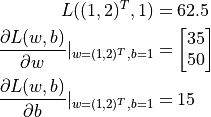## 基础示例：线性回归¶

 年份 2013 2014 2015 2016 2017 房价 12000 14000 15000 16500 17500

import numpy as np

X_raw = np.array([2013, 2014, 2015, 2016, 2017], dtype=np.float32)
y_raw = np.array([12000, 14000, 15000, 16500, 17500], dtype=np.float32)

X = (X_raw - X_raw.min()) / (X_raw.max() - X_raw.min())
y = (y_raw - y_raw.min()) / (y_raw.max() - y_raw.min())


• 初始化自变量为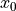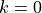• 迭代进行下列步骤直到满足收敛条件：

• 求函数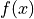关于自变量的梯度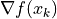• 更新自变量：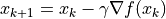。这里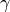是学习率（也就是梯度下降一次迈出的“步子”大小）

•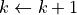### NumPy下的线性回归¶

a, b = 0, 0

num_epoch = 10000
learning_rate = 1e-3
for e in range(num_epoch):
# 手动计算损失函数关于自变量（模型参数）的梯度
y_pred = a * X + b

# 更新参数
a, b = a - learning_rate * grad_a, b - learning_rate * grad_b

print(a, b)


• 经常需要手工求函数关于参数的偏导数。如果是简单的函数或许还好，但一旦函数的形式变得复杂（尤其是深度学习模型），手工求导的过程将变得非常痛苦，甚至不可行。

### TensorFlow下的线性回归¶

TensorFlow的 Eager Execution（动态图）模式 5 与上述NumPy的运行方式十分类似，然而提供了更快速的运算（GPU支持）、自动求导、优化器等一系列对深度学习非常重要的功能。以下展示了如何使用TensorFlow计算线性回归。可以注意到，程序的结构和前述NumPy的实现非常类似。这里，TensorFlow帮助我们做了两件重要的工作：

• 使用 tape.gradient(ys, xs) 自动计算梯度；

• 使用 optimizer.apply_gradients(grads_and_vars) 自动更新模型参数。

X = tf.constant(X)
y = tf.constant(y)

a = tf.Variable(initial_value=0.)
b = tf.Variable(initial_value=0.)
variables = [a, b]

num_epoch = 10000
optimizer = tf.keras.optimizers.SGD(learning_rate=1e-3)
for e in range(num_epoch):
y_pred = a * X + b
loss = 0.5 * tf.reduce_sum(tf.square(y_pred - y))
# TensorFlow自动计算损失函数关于自变量（模型参数）的梯度
# TensorFlow自动根据梯度更新参数

print(a, b)


Python的 zip() 函数

zip() 函数是Python的内置函数。用自然语言描述这个函数的功能很绕口，但如果举个例子就很容易理解了：如果 a = [1, 3, 5]b = [2, 4, 6]，那么 zip(a, b) = [(1, 2), (3, 4), ..., (5, 6)] 。即“将可迭代的对象作为参数，将对象中对应的元素打包成一个个元组，然后返回由这些元组组成的列表”。在Python 3中， zip() 函数返回的是一个 zip 对象，本质上是一个生成器，需要调用 list() 来将生成器转换成列表。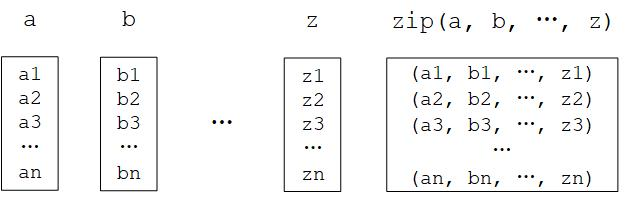Python的 zip() 函数图示

1

Python中可以使用整数后加小数点表示将该整数定义为浮点数类型。例如 3. 代表浮点数 3.0

2

3

4

5